# 110 年 - 110 國立高雄大學_碩士班招生考試_金融管理學系：統計學#102158

【非選題】
1.

1. Given n observed data points, say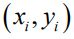for i = 1,2,3,..., n , please answer the following questions:

【題組】

(1). (10%) Please show that the straight line best fits the points is, where【非選題】
2.【題組】

(2). (3%) Given five observed data points in Table 1:Please use the results of (1) to find the least-squares line (regression line).

【非選題】
3.【題組】

(3). (5%) Please evaluate the coefficient of determination【非選題】
4.

2. Let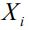, for i = 1, 2,3,... , be independent and identically distributed (i.i.d.) random variables. The expectation and variance of each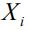are μ and σ2 , respectively. Please answer the following questions:

【題組】

(1). (5%) What is the probability distribution of?

【非選題】
5.【題組】

(2). (10%) Let x1 , x2 , x3 , …, xm be m samples of a random variable X . If m is sufficiently large ( m  30 ), please show that the 95% confidence interval for the mean of X is:whereanddenote the sample mean and sample standard deviation, respectively.

【非選題】
6.3. (10%) A teacher believes that the average grade of a class of 100 students is not higher than 85. The teacher randomly selects 64 students. The average grade of the 64 students is 87.8 and the standard deviation is 12.8. Using a significance level of 0.05, what conclusion should the teacher make?

【非選題】
7.

4. (5%) Letand Var ( X ) denote the expectation and variance of a random variable X , respectively. Please show that【非選題】
8.

5. Let X be normally distributed with mean 0 and variance 1. The probability density function of X is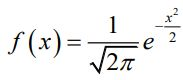. Please answer the following question:

【題組】

(1). (7%) What is the probability density function of, where μ and σ>0 are constant?

【非選題】
9.【題組】(2). (7%) What is the probability density function of X2 ?

【非選題】
10.

≤≤≤≤6. Let N(t) , where 0 ≤ t ≤ T , denote a stochastic process, which satisfies the following conditions:
i. N (0)= 0 .
ii. N (t2)- N (t1) is normally distributed with mean 0 and variance t2 - t1 , where
0 ≤ t1 < t2 ≤ T .

iii. N(t) has independent increments. That is, given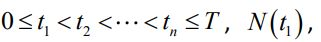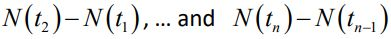are mutually independent.

【題組】 (1). (7%) Please evaluate the expectation of N(s)N(t) , where 0≤ s < t ≤ T . ( Hint: N(t)-N(s))+N(s)-N(0)))

【非選題】
11.【題組】

(2). (7%) Please show that the correlation coefficient between N(s) and N(t) is, where 0≤ s < t ≤ T .

【非選題】
12.【題組】

(3). (12%) Letdenote the price of a stock, where S ( 0 )= 10 is the share price at t = 0 . What is the expected share price at t = T ? That is, please evaluate the expectation of S(T) . ( Hint: N(t)=N(t)-N(0) is normally distributed.)

【非選題】
13.【題組】

(4). (12%) Let X =. Please show that the expectation of X isYou may begin with finding the minimum value of N(1) such that S(1)≥ 10 .